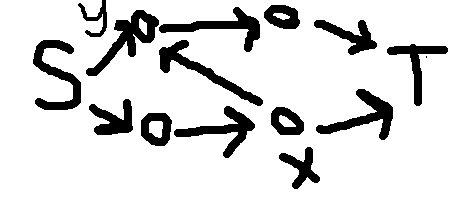# 一些算法（套路）

## 差分约束

如果只有 $a_i\leq a_j+d$ 的约束，就可以直接上差分约束。

如果有 $a_i+a_j\leq d$ 的约束，考虑整张图黑白染色，使得同色点之间只有差的约束，异色点之间只有和的约束，然后把白色的点的值取反，就可以跑差分约束了。

## 矩阵快速幂

观察一下矩阵是否是循环矩阵，如果是就可以用FFT解决（循环卷积）。

### 用BM算法优化矩阵快速幂DP

记 $B$ 为转移矩阵。

那么 $B$ 中每个元素都对应着同一个常系数线性递推关系。

证明：

记 $B$ 的特征多项式为 $f(x)=a_0x^k+a_1x^{k-1}+\cdots+a_kx^0$，那么就有

\begin{align} (a_0B^k+a_1B^{k-1}+\cdots+a_kB^0)&=0\\ B^t(a_0B^k+a_1B^{k-1}+\cdots+a_kB^0)&=0\\ a_0B^{k+t}&=-a_1B^{k-1+t}-a_2B^{k-2+t}-\cdots-a_kB^t\\ \end{align}

这样就可以求出前面 $O(k)$ 项然后 $O(k^2)$ BM一下得到递推式再用倍增取模在 $O(k^2\log n)$$O(k\log k\log n)$ 内求出任意项的值了。

如果要求整个矩阵的递推式的话，要拿这个矩阵哈希得到的值去BM，因为单项的最短递推式可能不是整个矩阵的最短递推式。

### 矩阵快速幂+DFT

DP转移如下：

$f_{i+1,j',k+v}+=f_{i,j,k}$

$i\leq n,j\leq l,k\leq m$
其中$v$只与$j$有关，最后求$k=s$$k\bmod m=s$的值的和。
暴力搞的时间复杂度是$O(l^3m^3\log n)$的。
我们可以把这个东西看成一个多项式。

$g_{i,j}=\sum_{k\geq 0}f_{i,j,k}x^k$

转移就可以看成乘以一个多项式（单项式）。
如果求的是$k\mod m=s$的值的和，就可以看成循环卷积。
可以先求值，把每个点值拿去跑一遍矩阵快速幂，再插值回来。
时间复杂度：$O(ml^3\log n)+$点值插值的时间复杂度$O(m^2)/O(m\log m)$

### 多组询问的矩阵快速幂优化DP

设矩阵大小为$m$，次数是$n$，询问组数是$t$，朴素的实现是$O(tm^3\log n)$的。
可以先把转移矩阵的$i$次幂求出来。
每次询问只需要拿一个$1\times m$的矩阵去乘转移矩阵就行了。每次乘法是$O(m^2)$的。
时间复杂度：$O(m^3\log n+tm^2\log n)$

## 带删除的线性基

对于线性基中的每个向量和所有 $0$ 向量维护这个向量是由哪些向量异或得到的。

在删除一个向量 $x$ 时，找到一个包含 $x$$0$ 向量，如果没有就找线性基里位最低的包含 $x$ 的向量，把这个向量的信息异或到其他包含 $x$ 的向量的信息中即可。这样在删除时不会影响线性基中更高位的向量。

在向量个数比较小或强制在线是比较有用。

## 排序

有些题如果把权值（或者其他东西）从小到大排序按顺序做，会有出人意料的效果。

## 定期重构

就每做 $O(\sqrt q)$ 个修改就重构一下，每次询问在建好的数据结构上查询，还要把剩下的 $O(\sqrt q)$ 的修改的影响一起算进去。

## 概率/期望DP

有一些概率/期望DP可以快速地推出这样的式子：

\begin{align} f_i&=a+bf_i\\ (1-b)f_i&=a\\ f_i&=\frac{a}{1-b} \end{align}

BZOJ4872

XSY2472

## 分治

有一些问题求得是只包含/不包含一个点的情况，只需要考虑当前$[l,r]$$[l,mid]$$[mid+1,r]$的影响。

下面来讲一道例题

$A(x)$$n-1$次多项式，$B_i(x)$为一次多项式，$\forall i$$A(x)\mod B_i(x)$

直接做是$O(n^2)$的。

因为$(A(x)\mod C(x))\mod B_i(x)=A(x)\mod B_i(x)$$C(x)\mod B_i(x)=0$

设当前已经求出了

$D_{l,r}=A(x)\mod(\prod_{i=l}^rB_i(x))$

那么

\begin{align} D_{l,mid}&=D_{l,r}\mod(\prod_{i=l}^{mid}B_i(x))\\ D_{mid+1,r}&=D_{l,r}\mod(\prod_{i=mid+1}^{r}B_i(x)) \end{align}

所以我们可以递归下去做，直到求出所有的$D_{i,i}$

时间复杂度：

$T(n)=2T(\frac{n}{2})+O(n\log n)=O(n\log^2n)$

多点求值

XSY2469

## 欧拉phi函数

就是$\varphi$函数

谁都知道这个东西是个积性函数。

$\varphi(ab)=\varphi(a)\varphi(b)~~~((a,b)=1)$

那如果$(a,b)\neq 1$呢？

设$d=(a,b)$

\begin{align} \varphi(a)&=a(1-\frac{1}{p1_1})(1-\frac{1}{p1_2})\cdots(1-\frac{1}{p1_{n1}})\\ \varphi(b)&=b(1-\frac{1}{p2_1})(1-\frac{1}{p2_2})\cdots(1-\frac{1}{p2_{n2}})\\ \varphi(ab)&=ab(1-\frac{1}{p3_1})(1-\frac{1}{p3_2})\cdots(1-\frac{1}{p3_{n3}})\\ \varphi(d)&=d(1-\frac{1}{p4_1})(1-\frac{1}{p4_2})\cdots(1-\frac{1}{p4_{n4}}) \end{align}

可以发现，对于后面那部分

\begin{align} (1-\frac{1}{p1_1})(1-\frac{1}{p1_2})\cdots(1-\frac{1}{p1_{n1}})\times (1-\frac{1}{p2_1})(1-\frac{1}{p2_2})\cdots(1-\frac{1}{p2_{n2}})\\ =(1-\frac{1}{p3_1})(1-\frac{1}{p3_2})\cdots(1-\frac{1}{p3_{n3}})\times (1-\frac{1}{p4_1})(1-\frac{1}{p4_2})\cdots(1-\frac{1}{p4_{n4}}) \end{align}

如果$p$只在$a$$b$中出现过，那么只会在$ab$中出现。如果同时在$a$$b$中出现过，那么会同时在$ab$$d$中出现。

所以有

$\varphi(ab)=\frac{\varphi(a)\varphi(b)d}{\varphi(d)}~~~(d=(a,b))$

## 逆向思维

### 情况一

有时候我们做某个操作很不好做，我们可以先把所有操作做完后在一个个回复。

例如：给以一个图，有两种操作：1.删边；2.询问连通性。

我们可以先把需要删的边删掉，再一个个加回来，用并查集维护连通性。

### 情况二

有时候问你$\forall A$，满足要求的$B$的和。

我们可以枚举所有的$B$，计算每个$B$对每个$A$的贡献。

AGC005F

## 一类全序问题

有$n$个物品，你要依次选择这些物品，每个物品有三个属性$a_i,b_i,c_i$，当你选择一个物品后，设当前选择的物品的$c$属性的和为$s$，那么选择这个物品的收益是$a_i+b_is$，问你最大收益是多少。

假设我们已经钦定了一个顺序。考虑两个相邻的物品（不妨设为前两个），什么时候当前顺序比交换后更优：

\begin{align} a_1+a_2+b_2c_1&>a_2+a_1+b_1c_2\\ b_2c_1&>b_1c_2\\ \frac{c_1}{b_1}&>\frac{c_2}{b_2} \end{align}

这样我们就得到了一个全序关系。

那么能不能扩展到任意两个物品的情况呢？

\begin{align} a_i+b_ix+a_j+b_j(x+y+c_i)&>a_j+b_jx+a_i+b_i(x+y+c_j)\\ b_jy+b_jc_i&>b_iy+b_ic_j\\ \frac{c_i+y}{b_i}&>\frac{c_j+y}{b_j}\\ \end{align}

好像并不太行。我们需要换一种思路。

假设我们找到了一种最优解，但并不满足以上的性质，那么一定可以交换相邻两个物品使得答案最优。所以直接排序贪心可以得到最优解。

如果题目还有其他限制，你也可以在得到这个顺序后DP或者干其他事情。

## 一类贪心问题

有 $n$ 个怪，组成了一棵树。

打一个怪会先扣 $a_i$ 滴血，在加 $b_i$ 滴血。

怪的父亲要在这个怪之前打。

问你初始时最少要有多少血才能把这个怪打完。

先不考虑父亲比儿子早选的限制，把所有怪排序。

考虑第一个怪，如果可以直接打，就直接打完。

否则这个怪会在打完父亲之后立刻打死，就把这个点和父亲合并。

如果要对每个子树求答案的话，可以用平衡树维护操作序列，自底向上合并所有点的操作序列。

## 莫队

总所周知，莫队的时间复杂度和块大小有关。

如果块大小为$t$，时间复杂度为$O(\frac{n^2}{t}+mt)$

如果块大小为$\sqrt n$，时间复杂度为$O((n+m)\sqrt n)$

如果块大小为$\frac{n}{\sqrt{m}}$，时间复杂度为$O(n\sqrt m)$

所以有时候可以通过调整块大小来加速。俗称调参。

## 一类单点修改区间求和的问题

有时候我们要修改一个点，求区间和。

树状数组/线段树的做法很经典，这里就不讲了。

分块1：每次修改把对应的位置和对应的块的和$+1$

分块2：每次修改把对应的位置和对应的块的和$+1$，然后求出块内前缀和、块内后缀和、块的前缀和。

有的人就要问了，分块做法那么慢，有什么用呢？

用处大着呢！当修改次数与询问次数不平衡的时候，我们可以做到比树状数组更优。

博主曾经用莫队+分块1水过了一道$n=m={10}^6$的题。跑的比zjt神犇的线段树合并还快。

## 和排列有关的问题

很多问题让你求对于每一个排列$A$，如果$\cdots$，那么$\cdots$

我们可以考虑从小到大插入这$n$个数，插入第$i$个数时考虑这个数的贡献。

## 用trie实现全部数$+1$，查询全部数的异或和

我们从低位到高位建一棵trie树。

从根开始，交换左右子树，然后对$0$的那棵子树执行同样的操作（进位）。

## 莫比乌斯反演

有很多题推着推着就推到$\varphi$上面去了。

说明可以用一条式子：$\sum_{d|n}\varphi(d)=n$

### 莫比乌斯反演的多组询问

\begin{align} ans&=\sum_{i=1}^lc^{\gcd(i,b)}\\ &=\sum_{d|s}c^d\sum_{i=1}^l[\gcd(i,s)=d]\\ &=\sum_{d|s}c^d\sum_{i=1}^{\frac{l}{d}}[\gcd(i,\frac{s}{d})=1]\\ &=\sum_{d|s}c^d\sum_{i|\frac{s}{d}}\mu(i)\lfloor\frac{l}{id}\rfloor\\ \end{align}

看起来没办法化简了

这时候要枚举$j=id$

\begin{align} ans&=\sum_{j|s}\lfloor\frac{l}{j}\rfloor\sum_{i|j}\mu(i)c^\frac{j}{i}\\ \end{align}

设$f(x)=\sum_{i|x}\mu(i)c^\frac{x}{i}$

这样$f(x)$就可以预处理出来了。

### 一般情况

\begin{align} ans&=\sum_{i=1}^n\sum_{j=1}^mf(\gcd(i,j))\\ &=\sum_{i=1}^{\min(n,m)}f(i)\sum_{i|j}\mu(\frac{j}{i})\lfloor\frac{n}{j}\rfloor\lfloor\frac{m}{j}\rfloor\\ &=\sum_{i=1}^{\min(n,m)}\lfloor\frac{n}{i}\rfloor\lfloor\frac{m}{i}\rfloor\sum_{j|i}f(j)\mu(\frac{i}{j}) \end{align}

这样可以预处理后面的$g(n)=\sum_{i|n}\mu(\frac{n}{i})f(i)$
每次枚举前面询问。
时间复杂度：$O(n+T\sqrt{n})$

## 分治FFT

分治FFT一般有两个用途。

### 求很多个多项式的乘积（普通分治）

设有$n$个多项式，次数之和是$m$，那么时间复杂度就是$O(m\log m\log n)$。一共有$\log n$层，每层是$O(m\log m)$的。

### 求一类数列（CDQ分治）

数列$f_n=\sum_{i=0}^{n-1}f_ig_{n-i}$。对于一个分治区间$[l,r]$，先求出$[l,mid]$的答案，再计算这部分对右边$[mid+1,r]$的贡献。

时间复杂度：$O(n\log^2 n)$

## 组合数学

$\sum_{i=j}^{n-k}\binom{i}{j}\binom{n-i}{k}=\binom{n+1}{j+k+1}$

可以用插板法理解。

## 树上的连通块

树上连通块个数$=$点数$-$边数。

点数/边数中一般有一个是固定的。

## 轻重链剖分&长链剖分

### 轻重链剖分

每个重链顶端的子树大小总和是 $O(n\log n)$ 的。

每个点到根经过的轻边个数是 $O(\log n)$ 的。

适用于维护与树的大小有关的信息。

### 长链剖分

每个长链顶端的子树深度总和是 $O(n)$ 的。

适用于维护与树的深度有关的信息。

## 高维前缀和 & 莫比乌斯反演

复杂度为 $O(\sum_{i}\frac{n}{p_i})=O(n\log log n)$

$g(n)=\sum_{d\mid n}f(d)$

for(int i=1;i<=cnt;i++)
for(int j=1;j*pri[i]<=n;j++)
f[j*pri[i]]+=f[j];


$g(n)=\sum_{n\mid d}f(d)$

for(int i=1;i<=cnt;i++)
for(int j=n/pri[i];j>=0;j--)
f[j]+=f[j*pri[i]];


$g(n)=\sum_{d\mid n}f(d)\mu(\frac{n}{d})$

for(int i=1;i<=cnt;i++)
for(int j=n/pri[i];j>=0;j--)
f[j*pri[i]]-=f[j];


$g(n)=\sum_{n\mid d}f(d)\mu(\frac{d}{n})$

for(int i=1;i<=cnt;i++)
for(int j=1;j*pri[i]<=n;j++)
f[j]-=f[j*pri[i]];


## 网络流

如果要的是最大收益，那么可以先强制选所有正的边，然后把不选一条边看成割掉这条边，答案为正的边权和 $-$ 最小割。

### 流量平衡

可以先对于每条边钦定一个方向，然后如果一条边有流量就表示这条边改了方向。对于一个点 $i$，要根据出度入度的关系向源/汇连边。

### 网格图

把每一行看做一个点，把每一列看做一个点，选一个格子就在对应的行列之间连边。

这是一个分层图，用 dinic 跑会比较快。

### 一种限制

每个物品对应一条链，割一条边代表这个物品对应的数/放在什么位置。

$i$ 对应的链割了点 $x$ 后面的边时 $j$ 对应的链必须割点 $y$ 后面的边

解决方法为连边 $(x,y,\infty)$有时候限制是 $i$ 割了点 $x$ 后面的边时 $j$ 必须割点 $y$ 前面的边

那么就要把一边的链反过来。

常见的方法有：

横着的链不变，竖着的链反过来。

黑白染色，白色的点对应的链不变，黑色的点对应的链反过来。

要注意前面的点属于 T 集且后面的点属于 S 集的情况。

要从后面的点往前面的点连容量为 $\infty$ 的边。

### 原图中不能有 $S\to T\to S$ 的路径

把所有反向边的容量设为 $\infty$ 即可。

## 点分树

二叉树的点分树中每个节点只有至多 $3$ 个儿子。这样可以直接可持久化这棵点分树，可以减少一个 $\log$（把点分树当成三叉数可持久化，深度是 $\log$ 的）。

如果题目给的树不是二叉树，可以强行转成二叉树。

## 最小树形图

用可合并堆维护每个点的最小入边，可以做到 $O(n+m)\log m$

## 容斥方法 总结

### 每次在剩下的物品中选一种拿走一个，求拿走的最后一个物品是第一种物品的概率 的问题

考虑容斥，枚举哪些物品是在第一种物品拿完之后拿走的（剩下的随意）。

那么剩下的物品就可以忽略了，只需要求出第一种物品是第一个拿完的概率。

对于 【UNR #3】百鸽笼 这道题，可以DP

考虑拿走第一种物品时每种物品拿了几个，就可以DP了：

设 $f_{i,j,k}$ 表示考虑完了前 $i$ 种物品，有 $j$ 种要在第一种取完之后才取完，已经取了 $k$ 个物品。

最终长度为 $l$ ，取了 $j$ 种的序列的序列的贡献是 方案数 $\times {(-1)}^j\times {(\frac{1}{j+1})}^l$

对于 【PKUWC2018】猎人杀 这道题，第一种物品是第一个取完的概率是 $\frac{w_1}{\sum w_i}$。可以用分治 NTT 计算方案数。

### 有 $m$ 种颜色的球排成一行，共 $n$ 个，求最终有 $k$ 个同色的球相邻的方案数

先假设每种球分成几段，然后用分治 FFT 算出方案数。

但是这样可能会有相邻且同色的段

这时候就可以容斥了。

考虑每种方案把相邻且同色的段合并之后有几段

那么就有

\begin{align} ans_i&=g_i−\sum{j<i}ans_{j}\binom{m−j}{i−j}\\ &=\sum_{j\leq i}g_j{(-1)}^{i-j} \end{align}

### 有 $m$ 种颜色的球排成一行，共 $n$ 个，最终贡献和同色段长度有关的

先算出每种长度的贡献

假设想分成 $i$ 段，但最终分成了 $j$ 段，那么此时的容斥系数是 ${(-1)}^{i-j}$，方案数是 $\binom{i-1}{j-1}$

然后拿最终分成的段数去跑 DP 就好了。

## 和树上两点间路径长度有关的技术&其他问题

比如说树上长度不超过 $k$ 的路径数量 等等

现在主要有三种方法：

### 长链剖分

这里讲几道题吧

LOJ571 $O(n\log^2n)$ $O(n\log^2n)$ $O(n\log n)$
[WC2010]重建计划 $O(n\log n\log V)$ $O(n\log n\log V)$ $O(n\log V)$

## ZKW 费用流中处理掉负权的方法

先用 SPFA 跑一边最短路，处理出 $S$ 到每个点的距离 $d_i$

显然对于每一条边 $(u,v,w)$ 都有 $d_u+w\geq d_v$

对于一条边 $(u,v,w)$，把这条边的边权变为 $w'=w+d_u-d_v$。这样整个图中就没有负权了。

容易证明在新图上跑出的最短路就是原图的最短路，只是长度有一点变化：$d'_T=0$

所以后面原点到汇点的距离要加上 $d_T$

## 用 dijkstra 代替 bellman-ford 跑费用流

还是先处理出 $S$ 到每个点的距离 $d_i$

还是把每条边的边权变为 $w'=w+d_u-d_v$

因为新建的反向边一定满足 $d_u+w_{u,v}=d_v$，所以 $w_{v,u}'=-w_{u,v}+d_v-d_u=0$

新图的最短路还是比原图的最短路少了 $d_T$

## 单位根反演（求和引理）

在碰到

$\sum_{i=0}^n\binom{n}{i}a^ib^{n-i}[k\mid i]$

时可以用求和引理优化：

\begin{align} [k\mid i]&=\frac{1}{k}\sum_{j=0}^{k-1}w_k^{ij}\\ &~~~~\sum_{i=0}^n\binom{n}{i}a^ib^{n-i}[k\mid i]\\ &=\sum_{i=0}^n\binom{n}{i}a^ib^{n-i}\frac{1}{k}\sum_{j=0}^{k-1}w_k^{ij}\\ &=\frac{1}{k}\sum_{j=0}^{k-1}\sum_{i=0}^n\binom{n}{i}a^ib^{n-i}w_k^{ij}\\ &=\frac{1}{k}\sum_{j=0}^{k-1}{(aw_k^j+b)}^n \end{align}

## prufer 序列

$K_{n,m}$ 的生成树个数是 $n^{m-1}m^{n-1}$

$K_{n_1,n_2,\ldots,n_k}$ 的生成树个数是 $n^{k-2}\prod_{i=1}^k{(n-n_i)}^{n_i-1}$，其中 $n=\sum_{i=1}^kn_i$

有一个 $n$ 个点，$m$ 个连通块，每个连通块大小为 $a_i$ 的森林。你要加上若干条边，让这个森林变成一棵树。方案数为

$\sum_{\sum_{i=1}^m d_i=2(m-1)}(m-2)!\prod_{i=1}^m\frac{a_i^{d_i}}{(d_i-1)!}\\ =n^{m-2}\prod_{i=1}^ma_i$

## 树形DP

$f_{i,j}$ 为以 $i$ 为根的子树，选出来的点数为 $j$ 时的方案数/贡献。这里 $j\leq k$

转移时要枚举两边各选了多少点。直接做是 $O(nk^2)$ 的。

注意到当选的点数 $\leq size$ 时才有意义。这样转移时两棵子树选的点数可以只枚举到 $\min(size,k)$，这样就是 $O(nk)$ 的了。

证明：
1.两棵子树大小都 $>k$。只有 $O(\frac{n}{k})$ 次转移，复杂度为 $O(\frac{n}{k}\times k^2)=O(nk)$
2.一棵子树大小 $\leq k$，另一颗子树大小 $>k$。对于所有的这类转移，小的那棵子树的大小之和是 $O(n)$ 的。复杂度为 $O(n\times k)=O(nk)$
3.两棵子树大小都 $\leq k$。把所有这类转移在树上标出来，那么会标出很多棵子树。每棵子树复杂度为 $O({size}^2)$，其中 $size\leq 2k$。复杂度为 $O(nk)$

这样总的复杂度就是 $O(nk)$ 了。

## 用全局平衡二叉树优化链剖+NTT

如果 $f_x$ 的次数 $x$ 子树的深度有关，就可以直接长链剖分+分治NTT做到 $O(n\log^2n)$

否则就要用重链剖分+分治NTT。复杂度为 $O(n\log^3n)$

但是我们可以在全局平衡二叉树上面合并。

一个点 $x$$size_{ls}\leq \frac{1}{2}size_x,size_{rs}\leq \frac{1}{2}size_x,\max(size_v)\leq \frac{1}{2}size_x$（这里 $v$$x$ 的轻儿子（虚儿子））。

这样只会跳 $O(\log n)$ 次实边。

那虚边呢？

如果是和子树深度有关的题，直接把 $f_v$ 加起来就好了。总复杂度为 $O(n\log^2n)$

如果是和子树大小有关的题，可以再用一次全局平衡二叉树的思想：找到一个点 $v$ 满足 $size_{ls}\leq \frac{1}{2}\sum size_v,size_{rs}\leq \frac{1}{2}\sum size_v$，但是中间那个儿子的 $size_y$ 可能会 $>\frac{1}{2}\sum size_v$。但这并不影响复杂度，因为 $size_y<\frac{1}{2}size_x$，所以从一个点的某个虚儿子 $v$ 跳到 $x$ 需要的步数是 $O(\log\frac{\sum size_v}{size _v}+1)=O(\log \frac{size_x}{size_v})$。所以总的复杂度就是 $O(n\log^2n)$

## DP优化

记 $[l_i,r_i]$$i\in g(k-1)$ 可以转移到的 $g(k)$ 中的区间（或者能转移到 $g(k)$ 的区间）。要求任意两个 $[l_i,r_i],[l_j,r_j]$ 不互相严格包含。（这里严格包含指的是包含且左端点不同且右端点不同）
我们可以把 $g(k)$ 切成若干个区间，满足 $[l_i,r_i]$ 不被任意一个区间严格包含，且 $[l_i,r_i]$ 最多与两个区间相交。
方法如下：对于一个区间的左端点，找到最右的右端点使得这个区间不严格包含任何 $[l_i,r_i]$。可以证明，这个方法满足条件。
这样，一个 $[l_i,r_i]$ 一定是一个区间的前缀或后缀，分两部分DP一下就好了。DP过程类似决策单调性优化DP。

## 区间加&区间 $\gcd$

$\gcd(a_1,a_2,\ldots,a_n)=\gcd(a_1,a_2-a_1,a_3-a_2,a_4-a_3,\ldots,a_n-a_{n-1})$

维护差分后的序列即可。

## 数位DP

有时候会遇到很多个数的和 $\leq m$，每个数 $\leq n$，还有一些其他限制的计数题。
可以从低位往高位数位DP，记录进位和只考虑低位的大小关系。

posted @ 2018-03-05 21:28  ywwyww  阅读(3780)  评论(4编辑  收藏